Home    |    Teacher    |    Parents    |    Glossary    |    About UsEmail this page to a friendResources· Cool Tools · Formulas & Tables · References · Test Preparation · Study Tips · Wonders of MathSearchDifferentiation Identities (Math | Calculus | Derivatives | Identities)

Definitions of the Derivative:
df / dx = lim (dx -> 0) (f(x+dx) - f(x)) / dx (right sided)
df / dx = lim (dx -> 0) (f(x) - f(x-dx)) / dx (left sided)
df / dx = lim (dx -> 0) (f(x+dx) - f(x-dx)) / (2dx) (both sided)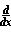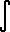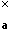f(t) dt = f(x) (Fundamental Theorem for Derivatives)c f(x) = c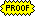f(x) (c is a constant)(f(x) + g(x)) =f(x) +g(x)f(g(x)) =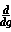f(g) *g(x) (chain rule)f(x)g(x) = f'(x)g(x) + f(x)g '(x) (product rule)f(x)/g(x) = ( f '(x)g(x) - f(x)g '(x) ) / g^2(x) (quotient rule)

### Partial Differentiation Identities

if f( x(r,s), y(r,s) )

df / dr = df / dx * dx / DR + df / dy * dy / DR
df / ds = df / dx * dx / Ds + df / dy * dy / Ds

if f( x(r,s) )

df / DR = df / dx * dx / DR
df / Ds = df / dx * dx / Ds

directional derivative

df(x,y) / d(Xi sub a) = f1(x,y) cos(a) + f2(x,y) sin(a)
(Xi sub a) = angle counterclockwise from pos. x axis.